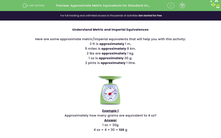# Understand Metric Equivalents for Standard Imperial Measures

In this worksheet, students revise changing imperial units to their approximate metric equivalents.Key stage:  KS 2

Curriculum topic:   Measurement

Curriculum subtopic:   Approximate Metric and Imperial Measurements

Difficulty level:#### Worksheet Overview

Understand Metric and Imperial Equivalences

Here are some approximate metric/imperial equivalents that will help you with this activity:

3 ft is approximately 1 m.
5 miles is approximately 8 km.
2 lbs are approximately 1 kg.
1 oz is approximately 30 g.
2 pints is approximately 1 litre.Example 1
Approximately how many grams are equivalent to 4 oz?

1 oz = 30g
4 oz = 4 × 30 = 120 g

Example 2
Approximately how many km are equivalent to 100 miles?

5 miles = 8 km
100 miles = 160 km (multiply each side by 20)

Now, let's put your understanding of approximate equivalences to the test.

Remember, you can view this introduction by clicking on the toolbar on the right at any time during this activity!

No calculators are permitted as we are calculating approximate equivalences, not exact equivalences.

### What is EdPlace?

We're your National Curriculum aligned online education content provider helping each child succeed in English, maths and science from year 1 to GCSE. With an EdPlace account you’ll be able to track and measure progress, helping each child achieve their best. We build confidence and attainment by personalising each child’s learning at a level that suits them.

Get started